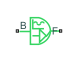# Sinusoidal Rotational Velocity Source

Produce sinusoidal rotational velocity

•Libraries:
Simscape / Driveline / Sources

## Description

The Sinusoidal Rotational Velocity Source block produces sinusoidal rotational velocity with a constant offset.

The relative rotational velocity between the base and follower interfaces is

`${v}_{FB}={v}_{F}-{v}_{B},$`

where:

• v FB is the relative rotational velocity.

• vF is the absolute rotational velocity at the follower interface.

• vB is the absolute rotational velocity at the base interface.

The defining equation for the sinusoidal rotational velocity that the block generates is

`$v={v}_{o}+A*\mathrm{sin}\left(2\pi ft+\phi \right),$`

where:

• v is the output rotational velocity.

• vo is the offset rotational velocity of the output.

• A is the peak amplitude of the sinusoid.

• f is frequency of the sinusoid in Hz.

• t is time.

• φ is phase shift of the sinusoid.

## Ports

### Conserving

expand all

Mechanical rotational conserving port associated with the base interface.

Mechanical rotational conserving port associated with the follower interface.

## Parameters

expand all

Average value that the output sinusoidal rotational velocity oscillates about.

Peak amplitude of the output sinusoidal rotational velocity.

Phase shift of the output sinusoidal rotational velocity.

Frequency of the output sinusoidal rotational velocity. The frequency must be greater than or equal to 0.

## Version History

Introduced in R2016b